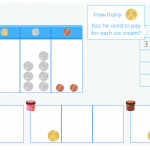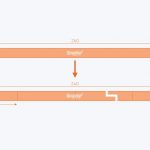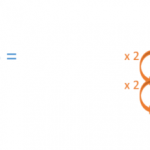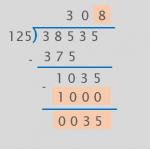Smartick is a fun way to learn math!# I want to learn about: Division

Content about division in elementary mathematics. Exercises, tutorials, problems, and teaching resources about division for elementary aged children. Here you will find posts about division to make learning mathematics easier and more fun.

Jul08

## Dividing with Decimals Using Money as an ExampleIn today’s post, you are going to learn how to solve division problems with decimals in the dividend, in other words, dividing a decimal number by a whole number. In order for you to understand the process well, I am going to show you how we explain dividing with decimals in the interactive Smartick tutorial […]

Sep17

## Practice Division with the Help of Everyday SituationsToday’s post about division is directed towards parents, to enhance their childs conception and processing in a practical way. Combined with their daily 15 minute math session on Smartick, I am sure they can perfectly understand division by the end of summer. Like every learning process, especially when referring to children, it is convenient to […]

Jul02

## The Relation between Multiplication and Division

Multiplication and division are closely related, given that division is the inverse operation of multiplication. When we divide, we look to separate into equal groups, while multiplication involves joining equal groups. In today’s post, we’re going to learn to use multiplication as a strategy to solve division problems, which will be really useful for daily life! We’ll start with a […]

May14

## Division Exercises Solved Using the Singapore MethodIn this post, we’re going a reference a previous one where we looked at the different types of division problems that exist according to how the problem is formulated. Taking these problems as examples, we’re going to look at different strategies to solve division exercises using the Singapore method. The first are division exercises involving repetition or equal […]

Jan26

## An Alternative Method to Help with DivisionIn today’s post we’re going to look at an alternative method of division. Schools often tend to teach the standard algorithm, but that’s not the only way of doing it. Once a child learns how to divide using the classic algorithm, there’s no need for them to use any alternative. The objective is to calculate the […]

Dec22

## Long Division: Divide by 3 Digit NumbersWe’ve already written various posts about dividing by 3 digits: Learn How to Divide with 3-Digit Numbers Practice 3-Digit Division Examples Learn How to Do a 3-Digit Division Problem Today we’re going to look at another, more complicated, example. Let’s start and divide by 3 digit numbers! 1. How many digits are there in the divisor? […]

Oct25

## What Is a Division? Basic Concepts

In today’s post we’re going to learn what a division is, and what we call the numbers that make up a division. Dividing is the same as sharing in equal parts For example: Imagine we have 10 cupcakes. We want to put them into 2 boxes so that each box has the same number of cupcakes. How […]

Aug11

## Strategy for Mental Calculation: HalvesIn this post we will learn a strategy to divide by 2 (halves), by 4 (fourths) or by 8 (eighths) mentally. We will start by looking at the concept of halving: Divide by Halves: To divide by 2, we just have to look for half of the number. For example, to divide 24/2, we find […]

Jul17

## Learn How to Solve 1-Digit Division ProblemsToday we are going to look at some examples on solving 1-digit division problems. In these types of problems, we need to divide numbers by a divisor that only has 1 digit. Before starting, feel free to click on the following links to review how to divide by 1-digit numbers: Division Word Problems With Decimals Ready?  Let’s […]